# Revision Notes For CBSE Class 10 Maths Chapter 4 Quadratic Equations

Quadratic equations are one of the main formulas to learn in class 10 maths. The equation is part of algebra and is basically a second-order polynomial equation in a single variable x. Thus, in chapter 4 of class 10 maths, students will be introduced to different quadratic equations and they will also learn to find solutions of quadratic equations either by factorisation or by completing the square method. While understanding and practising the use of this formula is very important, CBSE revision notes for class 10 maths chapter 4 – quadratic equations given here will help students do it in the right way. The notes have been designed to help students understand concepts clearly and at the same time develop better math skills. Students can also use these notes to prepare competently for the exams.

Students can glance through the class 10 maths chapter 4 CBSE revision notes PDF below.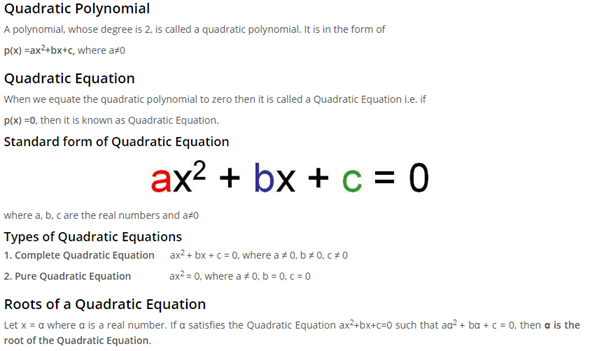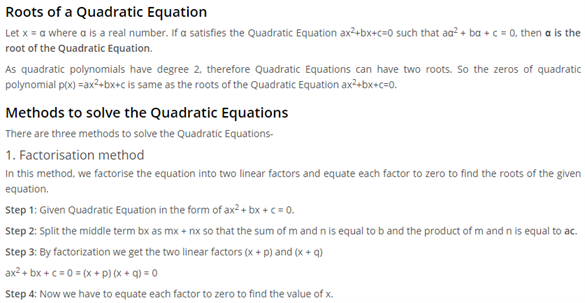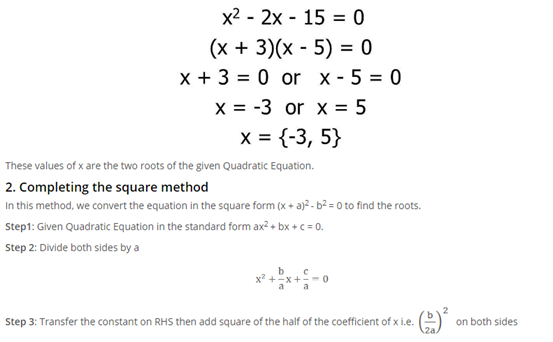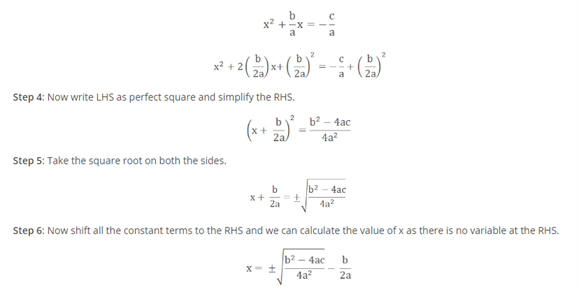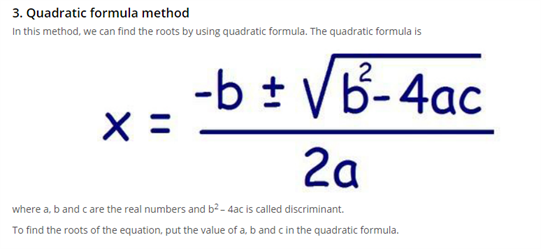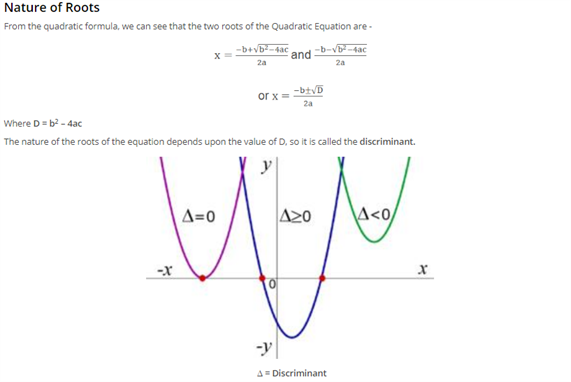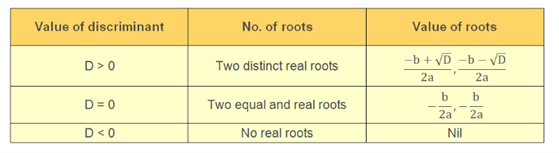Access CBSE Class 10 Maths Sample Papers Here.

Access NCERT Class 10 Maths Book Here.